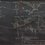# $n+2$ points define an $n$-sphere

Sometimes, it's fun to muse about basic stuff. I was in class and suddenly thought about how to prove the statement in the title.

Consider 4 points on the plane not coplanar. Choose 3, and construct the circumcircle. This will be a circle lying on our sphere. We may then construct an arbitrary sphere from this circle, and there are many methods for doing so (eg picking the radius) but we will go with the decidedly simplest method.

Choose $\theta \in (-\frac{\pi}{2},\frac{\pi}{2})$. Then create a cone by projecting rays from each point on the circle at an angle $\theta$, so that the rays converge on a point. A sphere is defined by its center and its radius, so the set of all spheres is isomorphic to $\mathbb{R}^3 \times \mathbb{R}^+$. The tip of the cone is the center, and the length from the center to any point on the circle is the radius.

Hence, we have a function $f: (-\frac{\pi}{2},\frac{\pi}{2}) \to \mathbb{R}^3 \times \mathbb{R}^+$. Since $\mathbb{R}^n$ is complete, $f$ is continuous and thus satisfies the intermediate value property. Eventually, the circle will pass through the fourth point, and since it will only pass through this point once, the sphere defined by our four points is unique.

By induction, this result can be extended to every n, as in the title.

This just goes to show the simultaneous power and rigour analysis can bring to the table.Note by Jake Lai
5 years, 4 months ago

This discussion board is a place to discuss our Daily Challenges and the math and science related to those challenges. Explanations are more than just a solution — they should explain the steps and thinking strategies that you used to obtain the solution. Comments should further the discussion of math and science.

When posting on Brilliant:

• Use the emojis to react to an explanation, whether you're congratulating a job well done , or just really confused .
• Ask specific questions about the challenge or the steps in somebody's explanation. Well-posed questions can add a lot to the discussion, but posting "I don't understand!" doesn't help anyone.
• Try to contribute something new to the discussion, whether it is an extension, generalization or other idea related to the challenge.
• Stay on topic — we're all here to learn more about math and science, not to hear about your favorite get-rich-quick scheme or current world events.

MarkdownAppears as
*italics* or _italics_ italics
**bold** or __bold__ bold
- bulleted- list
• bulleted
• list
1. numbered2. list
1. numbered
2. list
Note: you must add a full line of space before and after lists for them to show up correctly
paragraph 1paragraph 2

paragraph 1

paragraph 2

[example link](https://brilliant.org)example link
> This is a quote
This is a quote
    # I indented these lines
# 4 spaces, and now they show
# up as a code block.

print "hello world"
# I indented these lines
# 4 spaces, and now they show
# up as a code block.

print "hello world"
MathAppears as
Remember to wrap math in $$ ... $$ or $ ... $ to ensure proper formatting.
2 \times 3 $2 \times 3$
2^{34} $2^{34}$
a_{i-1} $a_{i-1}$
\frac{2}{3} $\frac{2}{3}$
\sqrt{2} $\sqrt{2}$
\sum_{i=1}^3 $\sum_{i=1}^3$
\sin \theta $\sin \theta$
\boxed{123} $\boxed{123}$

## Comments

There are no comments in this discussion.

×

Problem Loading...

Note Loading...

Set Loading...